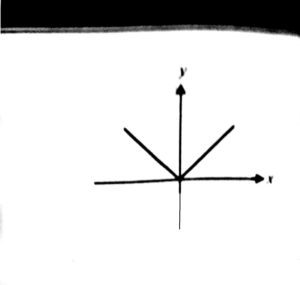IB Mathematics Tutors should give a fair number of hours in teaching functions. This is my third article on functions in the series of ib mathematics

For the sake of a comprehensive discussion, some standard functions and their graphs are discussed here

For the sake of a comprehensive discussion, some standard functions and their graphs are discussed here
1.Constant Function-
F(x)=k
Domain= ℝ

Range    = {k}

Range    = {k}

2.Identity Function-

F(x)=x
Domain=
Range   =

3.Linear Function-

F(x)=mx+c
Domain=  ℝ

Range   =

F(x)=x2
Domain=  ℝ

Range    =(0, ∞]

5.Cube Function-

F(x)=x3
Domain=

Range    =

6.Reciprocal Function-

F(x)=1/x
Domain=   ℝ-{0}

Range    = ℝ-{0}

7.Step Function-

F(x)=0    if   x∠0

=1   if   x≥0
Domain=

Range    ={0,1}

8. Absolute value Function-

F(x)=|x|     = x    if x≥0

=-x  if x∠0
Basically, this function gives the magnitude of a number and strips it of its negative sign, if it is negative.
Domain=
Range   = [0, ∞)9.Exponential Function-

F(x)=  ex
Domain=  ℝ

Range    =(0,∞)

10.Logarithmic Function-

F(x)=lnx
Range     =

Domain   = (0,∞)

11.Exponential Function-

F(x)=  ex
Domain=

Range    =(0,∞)

Notice what happen if we draw F(x)=ex and F(x)=lnx on the same axis
the graph of each function seems to be mirror reflections of each other in the line y=x
(In fact, they are!!)